PHY294H - Lecture 30

RL circuits, DC source

Kirchhoff's loop law for a circuit containing a DC voltage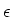, a resistor R and an inductor L is,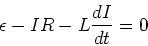(1)

The solution to this equation is,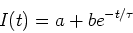(2)

where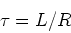(3)

The constants a and bdepend on the initial conditions.

Initial current is zero

In this case, we imagine that a switch is in the circuit and that the switch is open for t<0. We thus have I(0) = 0. This implies that

 a + b = 0 (4)

Since there is no current at time t=0, the voltage across the resistor is zero at t=0. Therefor the entire voltage is dropped across the inductor. We thus have,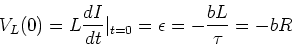(5)

From this equation we find,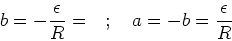(6)

The current in the circuit is then,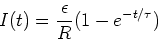(7)

At long times(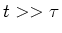), the inductance plays no role in this DC circuit.

Initial current is I0, no applied voltage

In this case we have,

 I(0) = I0 = a + b (8)

We also have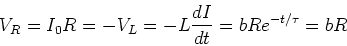(9)

We then have,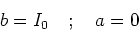(10)

so that,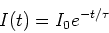(11)

An LC circuit - an oscillator

A circuit with an inductor and a capacitor acts as an oscillator. There is no dissipation in this circuit, provided the capacitor and the inductor are ideal.

We shall consider a case in which the capacitor has charge Q0 at t=0 and that at this time there is no current flowing in the circuit. The capacitor at time t=0 stores energy Q02/C, while the inductor stores no energy at t=0. At time t=0, we then have,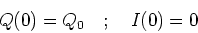(12)

As time goes on, energy is exhanged between the capacitor and the inductor. This is very similar to the exchange between kinetic and potential energy in a spring-mass system or in a pendulum.

Kirchhoff's loop law for the LC ciruit is,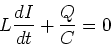(13)

Since I=dQ/dt, this equation is equivalent to,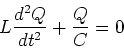(14)

This is the equation for simple harmonic motion and has the general solution,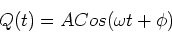(15)

where A and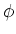are constants which we may choose to satisfy the initial conditions. In our example, the initial conditions are, Q(0) = Q0 and I(0) = 0, which are satisfied by,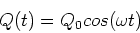(16)

LRC circuit

We are just adding a resistor to the problem we solved above. Kirchhoff's loop law gives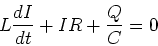(17)

Using I=dQ/dt we find,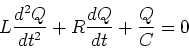(18)

The general solution to this equation is,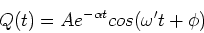(19)

where A andare constants which are chosen to fit the initial conditions.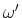and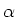are found by subsituting Eq. (19) into equation (18) and ensuring that Eq. (19) is a solution for all time. To carry out this calculations, we need to take derivatives of the charge with the respect to time. The first derivative is,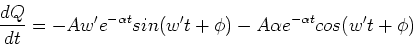(20)

and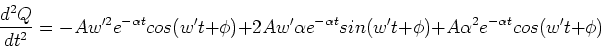(21)